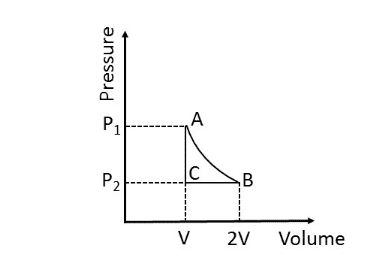# Net heat in a thermodynamic cycle

• Kaguro

#### Kaguro

Homework Statement
In the thermodynamic cycle shown, one mole of monoatomic ideal gas is taken through the cycle. AB is a reversible isothermal expansion at a temperature of 800K in which the volume of the gas is doubled. BC is an isobaric contraction to the original volume in which the temperature is reduced to 300K. CA is a constant volume process in which the pressure and temperature return to their initial values. Find the net amount of heat absorbed .
Relevant Equations
PV=RT
Q=C(T2-T1)
dU=dQ-dW
Cv=1.5R for monoatomic ideal gas
Cp = Cv+R = 2.5RIn AB, dU=0 (Isothermal)
Hence dQ=dW
W1= RT ln(2V/V) = RT ln(2) = 800R*ln(2)
Q1=W1 = 800R*ln(2)

Q2= Cp(300-800)=-500Cp = -500*2.5R

Q3 = Cv(800-300) = 500Cv = 500*1.5R

So Q= 800R*ln(2) -500*2.5R + 500*1.5R = 800R*ln(2) -500R
Hence net heat absorbed is 800R*ln(2) -500R

But.

Suppose I find work done instead:

W1 = 800R*ln(2)
W2 = P2(ΔV) = P2*(V-2V)=-P2*V
W3=0

Now, P1V1=RT
P2V2 = RT
So, P2=P1/2=0.5P1

So,W2=-0.5*P1*V1=-0.5*R*T1 = -400R

So, Net work done is 800*R*ln(2) - 400R
As this is a cycle, dU=0 over it. And net heat absorbed is net work done. Hence
Net heat absorbed is 800*R*ln(2) - 400R

There is inconsistency here. What did I do wrong?

The 300 K is inconsistent with the pressure being half the original pressure at point C. It should be 400 K.

•KaguroI didn't notice that..

It's very annoying to have national level entrance exams having wrong questions.Thanks very much for pointing it out.